Code for all the examples is located in your PYTHONPATH/Lib/site-packages/eonr/examples folder. With that said, you should be able to make use of EONR by following and executing the commands in this tutorial using either the sample data provided or substituting in your own data.

You will find the following code included in the advanced_tutorial.py or advanced_tutorial.ipynb (for Jupyter notebooks) files in your PYTHONPATH/Lib/site-packages/eonr/examples folder - feel free to load that into your Python IDE to follow along.

## 3.2. Calculate EONR for several economic scenarios¶

In this tutorial, we will run EONR.calculate_eonr() in a loop, adjusting the economic scenario prior to each run.

Load pandas and EONR:

:

import os
import pandas as pd
import eonr
print('EONR version: {0}'.format(eonr.__version__))

EONR version: 0.2.0


EONR uses Pandas dataframes to access and manipulate the experimental data.

:

df_data = pd.read_csv(os.path.join('data', 'minnesota_2012.csv'))
df_data

:

year location plot trt rep time_n rate_n_applied_kgha yld_grain_dry_kgha nup_total_kgha soil_plus_fert_n_kgha
0 2012 Minnesota 101 8 1 Pre 235.3785 12410.916200 198.759898 284.69590
1 2012 Minnesota 102 3 1 Pre 67.2510 10627.946000 147.971755 116.56840
2 2012 Minnesota 103 1 1 Pre 0.0000 7428.081218 98.769392 38.10890
3 2012 Minnesota 104 2 1 Pre 33.6255 9202.953180 111.440210 71.73440
4 2012 Minnesota 105 4 2 Pre 100.8765 10841.127180 142.663887 154.67730
5 2012 Minnesota 106 7 2 Pre 201.7530 10646.649330 178.802092 255.55380
6 2012 Minnesota 107 6 2 Pre 168.1275 12367.436000 186.053531 201.75300
7 2012 Minnesota 108 5 2 Pre 134.5020 13366.361700 196.737290 168.12750
8 2012 Minnesota 201 7 1 Pre 201.7530 14232.053480 228.775204 251.07040
9 2012 Minnesota 202 5 1 Pre 134.5020 14384.824980 226.006218 183.81940
10 2012 Minnesota 203 6 1 Pre 168.1275 13592.219290 182.423028 206.23640
11 2012 Minnesota 204 4 1 Pre 100.8765 14091.078390 187.745096 138.98540
12 2012 Minnesota 205 3 2 Pre 67.2510 10739.981390 133.470950 121.05180
13 2012 Minnesota 206 1 2 Pre 0.0000 8375.090921 109.460245 53.80080
14 2012 Minnesota 207 8 2 Pre 235.3785 13797.485850 195.932161 269.00400
15 2012 Minnesota 208 2 2 Pre 33.6255 9713.487469 113.903035 67.25100
16 2012 Minnesota 301 3 3 Pre 67.2510 12579.012170 180.812783 106.48075
17 2012 Minnesota 302 7 3 Pre 201.7530 13604.571780 208.724988 240.98275
18 2012 Minnesota 303 2 3 Pre 33.6255 10185.959390 121.505528 68.37185
19 2012 Minnesota 304 6 3 Pre 168.1275 14305.321460 204.391319 202.87385
20 2012 Minnesota 305 8 4 Pre 235.3785 13929.592020 186.775288 267.88315
21 2012 Minnesota 306 4 4 Pre 100.8765 10975.799250 147.057081 133.38115
22 2012 Minnesota 307 5 4 Pre 134.5020 11338.070290 148.348790 162.52325
23 2012 Minnesota 308 1 4 Pre 0.0000 5821.373521 68.791363 28.02125
24 2012 Minnesota 401 5 3 Pre 134.5020 13755.002370 198.844611 173.73175
25 2012 Minnesota 402 1 3 Pre 0.0000 9077.628329 122.207140 39.22975
26 2012 Minnesota 403 4 3 Pre 100.8765 13760.323240 181.734857 135.62285
27 2012 Minnesota 404 8 3 Pre 235.3785 14896.886860 227.454749 270.12485
28 2012 Minnesota 405 2 4 Pre 33.6255 10551.466010 144.282839 66.13015
29 2012 Minnesota 406 6 4 Pre 168.1275 15621.406530 227.056199 200.63215
30 2012 Minnesota 407 3 4 Pre 67.2510 10950.450720 146.628851 95.27225
31 2012 Minnesota 408 7 4 Pre 201.7530 13838.222530 210.340625 229.77425

## 3.5. Set column names and units¶

The table containing the experimental data must have a minimum of two columns:

• Nitrogen fertilizer rate

• Grain yield

We’ll also set nitrogen uptake and available nitrogen columns right away for calculating the socially optimum nitrogen rate.

As a reminder, we are declaring the names of these columns and units because they will be passed to EONR later.

:

col_n_app = 'rate_n_applied_kgha'
col_yld = 'yld_grain_dry_kgha'
col_crop_nup = 'nup_total_kgha'
col_n_avail = 'crop_n_available_kgha'

unit_currency = '$' unit_fert = 'kg' unit_grain = 'kg' unit_area = 'ha' def calc_mineralization(df_data, units_fert='kgha'): ''' Calculates mineralization and adds "crop_available_n" to df ''' df_trt0 = df_data[df_data['rate_n_applied_kgha']==0].copy() df_trt0['mineralize_n'] = (df_trt0['nup_total_kgha'] - df_trt0['soil_plus_fert_n_kgha']) trt0_mineralize = df_trt0['mineralize_n'].mean() crop_n_label = 'crop_n_available_' + units_fert df_data[crop_n_label] = df_data['soil_plus_fert_n_kgha'] + trt0_mineralize return df_data df_data = calc_mineralization(df_data)  ## 3.6. Turn base_zero off¶ You might have noticed the base_zero option for the EONR class in the API. base_zero is a True/False flag that determines if gross return to nitrogen should be expressed as an absolute values. We will see a bit later that upon executing EONR.calculate_eonr(), grain yield from the input dataset is used to create a new column for gross return to nitrogen (“grtn”) by multiplying the grain yield column by the price of grain (price_grain variable). If base_zero is True (default), the observed yield return data are standardized so that the best-fit quadratic-plateau model passes through the y-axis at zero. This is done in two steps: 1. Fit the quadratic-plateau to the original data to determine the value of the y-intercept of the model ($$\beta_0$$) 2. Subtract $$\beta_0$$ from all data in the recently created “grtn” column (temporarily stored in EONR.df_data) This behavior (base_zero = True) is the default in EONR. However, base_zero can simply be set to False during the initialization of EONR. We will set it store it in its own variable now, then pass to EONR during initialization: :  base_zero = False  ## 3.7. Initialize EONR¶ Let’s set the base directory and initialize an instance of EONR, setting cost_n_fert = 0, costs_fixed = 0, and price_grain = 1.0 (\$1.00 per kg) as the default values (we will adjust them later on in the tutorial):

:

import os

my_eonr = eonr.EONR(cost_n_fert=0,
costs_fixed=0,
price_grain=1.0,
col_n_app=col_n_app,
col_yld=col_yld,
col_crop_nup=col_crop_nup,
col_n_avail=col_n_avail,
unit_currency=unit_currency,
unit_grain=unit_grain,
unit_fert=unit_fert,
unit_area=unit_area,
base_dir=base_dir,
base_zero=base_zero)


## 3.8. Calculate the AONR¶

You may be wondering why cost_n_fert was set to 0. Well, setting our nitrogen fertilizer cost to $0 essentially allows us to calculate the optimum nitrogen rate ignoring the cost of the fertilizer input. This is known as the Agronomic Optimum Nitrogen Rate (AONR). The AONR provides insight into the maximum achievable grain yield. Notice price_grain was set to 1.0 - this effectively calculates the AONR so that the maximum return to nitrogen (MRTN), which will be expressed as$ per ha when ploting via EONR.plot_eonr(), is similar to units of kg per ha (the units we are using for grain yield).

Let’s calculate the AONR and plot it (adjusting y_max so it is greater than our maximum grain yield):

:

my_eonr.calculate_eonr(df_data)
my_eonr.plot_eonr(x_min=-5, x_max=300, y_min=-100, y_max=18000)


Computing AONR for Minnesota 2012 Pre
Cost of N fertilizer: $0.00 per kg Price grain:$1.00 per kg
Fixed costs: $0.00 per ha Agronomic optimum N rate (AONR): 177.4 kg per ha [139.9, 234.0] (90.0% confidence) Maximum return to N (MRTN):$13579.23 per ha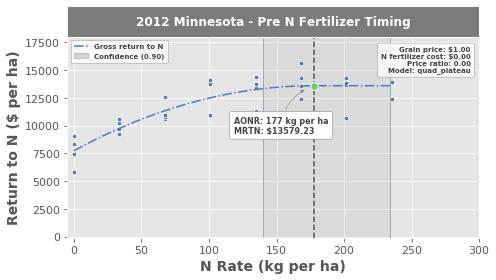We see that the agronomic optimum nitrogen rate was calculated as 177 kg per ha, and the MRTN is 13.579 Mg per ha (yes, it says $13,579, but because price_grain was set to \$1, the values are equivalent and the units can be substituted.

If you’ve gone through the first tutorial, you’ll notice there are a few major differences in the look of this plot:

The red line representing nitrogen fertilizer cost is missing

The GRTN line does not pass through the y-intercept at $$\text{y}=0$$?

Because base_zero was set to False, the observed data (blue points) were not standardized as to “force” the best-fit model from passing through at $$\text{y}=0$$.

## 3.9. Bootstrap confidence intervals¶

We will calculate the AONR again, but this time we will compute the bootstrap confidence intervals in addition to the profile-likelihood and Wald-type confidence intervals. To tell EONR to compute the bootstrap confidence intervals, simply set bootstrap_ci to True in the EONR.calcualte_eonr() function:

:

my_eonr.calculate_eonr(df_data, bootstrap_ci=True)
my_eonr.plot_tau()
my_eonr.fig_tau = my_eonr.plot_modify_size(fig=my_eonr.fig_tau.fig, plotsize_x=5, plotsize_y=4.0)


Computing AONR for Minnesota 2012 Pre
Cost of N fertilizer: $0.00 per kg Price grain:$1.00 per kg
Fixed costs: $0.00 per ha Agronomic optimum N rate (AONR): 177.4 kg per ha [139.9, 234.0] (90.0% confidence) Maximum return to N (MRTN):$13579.23 per ha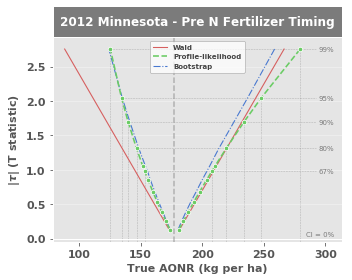## 3.10. Set fixed costs¶

Fixed costs (on a per area basis) can be considered by EONR. Simply set the fixed costs (using EONR.update_econ()) before calculating the EONR:

:

costs_fixed = 12.00  # set to $12 per hectare my_eonr.update_econ(costs_fixed=costs_fixed)  ## 3.11. Loop through several economic conditions¶ EONR computes the optimum nitrogen rate for any economic scenario that we define. The EONR class is designed so the economic conditions can be adjusted, calculating the optimum nitrogen rate after each adjustment. We just have to set up a simple loop to update the economic scenario (using EONR.update_econ()) and calculate the EONR (using EONR.calculate_eonr()). We will also generate plots and save them to our base directory right away: :  price_grain = 0.157 # set from$1 to 15.7 cents per kg
cost_n_fert_list = [0.44, 1.32, 2.20]

for cost_n_fert in cost_n_fert_list:
# first, update fertilizer cost
my_eonr.update_econ(cost_n_fert=cost_n_fert,
price_grain=price_grain)
# second, calculate EONR
my_eonr.calculate_eonr(df_data)
# third, generate (and save) the plots
my_eonr.plot_eonr(x_min=-5, x_max=300, y_min=-100, y_max=2600)
my_eonr.plot_save(fname='eonr_mn2012_pre.png', fig=my_eonr.fig_eonr)


Computing EONR for Minnesota 2012 Pre
Cost of N fertilizer: $0.44 per kg Price grain:$0.16 per kg
Fixed costs: $12.00 per ha Economic optimum N rate (EONR): 169.9 kg per ha [135.2, 220.9] (90.0% confidence) Maximum return to N (MRTN):$2043.53 per ha

Computing EONR for Minnesota 2012 Pre
Cost of N fertilizer: $1.32 per kg Price grain:$0.16 per kg
Fixed costs: $12.00 per ha Economic optimum N rate (EONR): 154.8 kg per ha [125.7, 195.0] (90.0% confidence) Maximum return to N (MRTN):$1900.67 per ha

Computing EONR for Minnesota 2012 Pre
Cost of N fertilizer: $2.20 per kg Price grain:$0.16 per kg
Fixed costs: $12.00 per ha Economic optimum N rate (EONR): 139.7 kg per ha [115.9, 170.1] (90.0% confidence) Maximum return to N (MRTN):$1771.10 per ha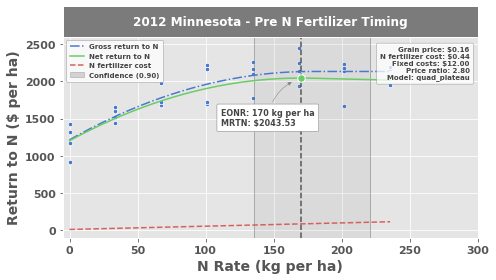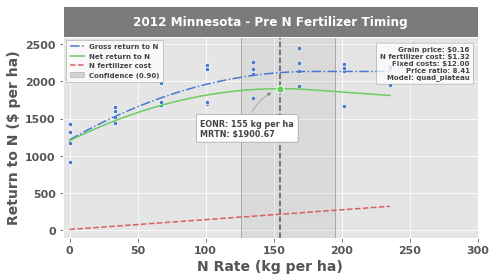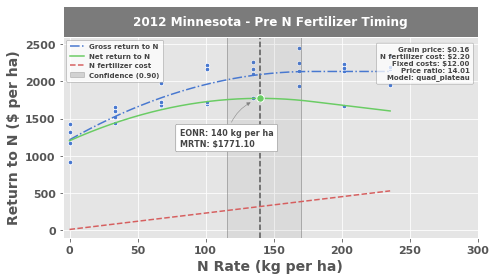A similar loop can be made adjusting the social cost of nitrogen. my_eonr.base_zero is set to True to compare the graphs:

:

price_grain = 0.157  # keep at 15.7 cents per kg
cost_n_fert = 0.88  # set to be constant
my_eonr.update_econ(price_grain=price_grain,
cost_n_fert=cost_n_fert)
my_eonr.base_zero = True  # let's use base zero to compare graphs

cost_n_social_list = [0.44, 1.32, 2.20, 4.40]

for cost_n_social in cost_n_social_list:
# first, update social cost
my_eonr.update_econ(cost_n_social=cost_n_social)
# second, calculate EONR
my_eonr.calculate_eonr(df_data)
# third, generate (and save) the plots
my_eonr.plot_eonr(x_min=-5, x_max=300, y_min=-400, y_max=1400)
my_eonr.plot_save(fname='eonr_mn2012_pre.png', fig=my_eonr.fig_eonr)


Computing SONR for Minnesota 2012 Pre
Cost of N fertilizer: $0.88 per kg Price grain:$0.16 per kg
Fixed costs: $12.00 per ha Social cost of N:$0.44 per kg
Socially optimum N rate (SONR): 160.1 kg per ha [129.0, 204.0] (90.0% confidence)
Maximum return to N (MRTN): $749.35 per ha Computing SONR for Minnesota 2012 Pre Cost of N fertilizer:$0.88 per kg
Price grain: $0.16 per kg Fixed costs:$12.00 per ha
Social cost of N: $1.32 per kg Socially optimum N rate (SONR): 155.8 kg per ha [126.3, 196.7] (90.0% confidence) Maximum return to N (MRTN):$737.04 per ha

Computing SONR for Minnesota 2012 Pre
Cost of N fertilizer: $0.88 per kg Price grain:$0.16 per kg
Fixed costs: $12.00 per ha Social cost of N:$2.20 per kg
Socially optimum N rate (SONR): 151.8 kg per ha [123.8, 189.9] (90.0% confidence)
Maximum return to N (MRTN): $725.79 per ha Computing SONR for Minnesota 2012 Pre Cost of N fertilizer:$0.88 per kg
Price grain: $0.16 per kg Fixed costs:$12.00 per ha
Social cost of N: $4.40 per kg Socially optimum N rate (SONR): 142.8 kg per ha [117.9, 175.0] (90.0% confidence) Maximum return to N (MRTN):$701.67 per ha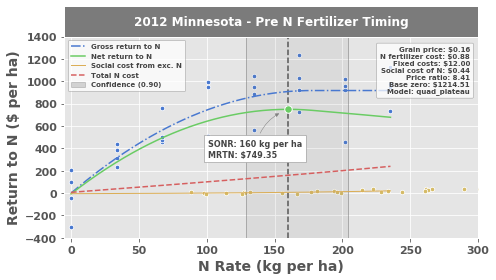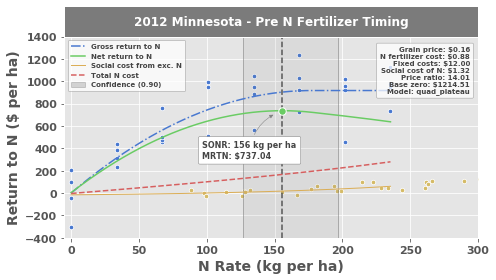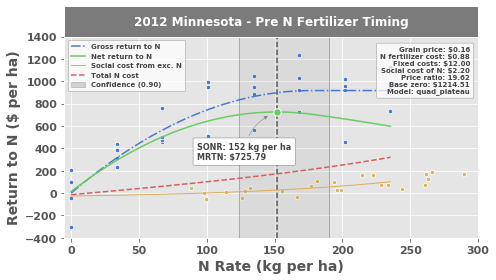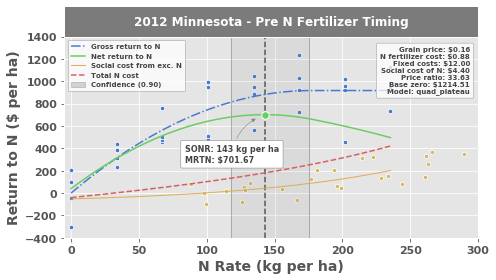Notice that we used the same EONR instance (my_eonr) for all runs. This is convenient if there are many combinations of economic scenarios (or many experimental datasets) that you’d like to loop through. If you’d like the results to be saved separate (perhaps to separate results depending if a social cost is considered) that’s fine too. Simply create a new instance of EONR and customize how you’d like.

## 3.12. View results¶

All eight runs can be viewed in the dataframe:

:

my_eonr.df_results

:

price_grain cost_n_fert cost_n_social costs_fixed price_ratio unit_price_grain unit_cost_n location year time_n ... mrtn grtn_r2_adj grtn_rmse grtn_max_y grtn_crit_x grtn_y_int scn_lin_r2 scn_lin_rmse scn_exp_r2 scn_exp_rmse
0 1.000 0 0.00 0 0.000000 $per ha$ per kg Minnesota 2012 Pre ... 13579.232456 0.728926 1154.305686 13579.232456 177.437339 7735.725352 None None None None
1 1.000 0 0.00 0 0.000000 $per ha$ per kg Minnesota 2012 Pre ... 13579.232456 0.728926 1154.305686 13579.232456 177.437339 7735.725352 None None None None
2 0.157 0.44 0.00 12 2.802548 $per ha$ per kg Minnesota 2012 Pre ... 2043.528038 0.728926 181.225993 2131.939496 177.437340 1214.508882 None None None None
3 0.157 1.32 0.00 12 8.407643 $per ha$ per kg Minnesota 2012 Pre ... 1900.670950 0.728926 181.225993 2131.939496 177.437340 1214.508882 None None None None
4 0.157 2.2 0.00 12 14.012739 $per ha$ per kg Minnesota 2012 Pre ... 1771.101634 0.728926 181.225993 2131.939496 177.437340 1214.508882 None None None None
5 0.157 0.88 0.44 12 8.407643 $per ha$ per kg Minnesota 2012 Pre ... 749.347111 0.728926 181.225993 917.433580 177.439985 0.002964 0.777106 55.7255 0.836099 8.44737
6 0.157 0.88 1.32 12 14.012739 $per ha$ per kg Minnesota 2012 Pre ... 737.042610 0.728926 181.225993 917.433580 177.439985 0.002964 0.777106 167.176 0.836099 25.3421
7 0.157 0.88 2.20 12 19.617834 $per ha$ per kg Minnesota 2012 Pre ... 725.792236 0.728926 181.225993 917.433580 177.439985 0.002964 0.777106 278.627 0.836099 42.2368
8 0.157 0.88 4.40 12 33.630573 $per ha$ per kg Minnesota 2012 Pre ... 701.669696 0.728926 181.225993 917.433580 177.439985 0.002964 0.777106 557.255 0.836099 84.4737

9 rows × 33 columns

EONR.df_results contains the following data columns (some columns are hidden by Jupyter in the table above):

• price_grain – price of grain

• cost_n_fert – cost of nitrogen fertilizer

• cost_n_social – other “social” costs of nitrogen

• price_ratiocost:grain price ratio

• unit_price_grain – units descirbing the price of grain

• unit_cost_n – units describing cost of nitrogen (both fertilizer and social costs)

• location – location of dataset

• year – year of dataset

• time_n – nitrogen application timing of dataset

• model – the model used to fit the experimental data (e.g., “quadratic” or “quad_plateau”).

• base_zero – if base_zero = True, this is the y-intercept ($$\beta_0$$) of the quadratic-plateau model before standardizing the data

• eonr – optimum nitrogen rate (can be agronomic, economic, or socially optimum; in units of EONR.unit_nrate)

• eonr_bias – bias in reparameterized quadratic-plateau model for computation of confidence intervals

• R* – the coefficient representing the generalized cost function

• costs_at_onr – total costs at the optimum nitrogen rate

• ci_level – confidence interval (CI) level (for subsequent confidence bounds)

• ci_wald_l – lower Wald CI

• ci_wald_u – upper Wald CI

• ci_pl_l – lower profile-likelihood CI

• ci_pl_u – upper profile-likelihood CI

• ci_boot_l – lower bootstrap CI

• ci_boot_u – upper bootstrap CI

• mrtn – maximum return to nitrogen (in units of EONR.unit_currency)

• grtn_r2_adj – adjusted $$\text{r}^2$$ value of the gross return to nitrogen (GRTN) model

• grtn_rmse – root mean squared error of the GRTN

• grtn_max_y – maximum y value of the GRTN (in units of EONR.unit_rtn)

• grtn_crit_x – critical x-value of the GRTN (point where the “quadratic” part of the quadratic-plateau model terminates and the “plateau” commences)

• grtn_y_int – y-intercept ($$\beta_0$$) of the GRTN model

• scn_lin_r2 – adjusted $$\text{r}^2$$ value of the linear best-fit for social cost of nitrogen

• scn_lin_rmse – root mean squared error of the the linear best-fit for social cost of nitrogen

• scn_exp_r2 – adjusted $$\text{r}^2$$ value value of the exponential best-fit for social cost of nitrogen

• scn_exp_rmse – root mean squared error of the the exponential best-fit for social cost of nitrogen

## 3.13. Save the data¶

The results generated by EONR can be saved to the EONR.base_dir using the Pandas df.to_csv() function. A folder will be created in the base_dir whose name is determined by the current economic scenario of my_eonr (in this case “social_336_4400”, corresponding to cost_n_social > 0, price_ratio == 33.6, and cost_n_social == 4.40 for “social”, “336”, and “4400” in the folder name, respectively):

:

print(my_eonr.base_dir)


F:\nigo0024\Documents\GitHub\eonr\eonr\examples\eonr_advanced_tutorial\social_336_4400